E-ISSN 1658-7073 | ISSN 1658-66382020, Vol: 7, Issue: 1

Article Format
Author Declaration

Original Research

Solving Satisfiability Logic Programming Using Radial Basis Function Neural Networks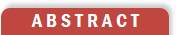Abstract
We proposed a new technique to solve QBF based on Radial basis function neural networks (RBFNNs) and Prey-Predator algorithm (PPA). Prey-Predator algorithm (PPA) is a neural learning algorithm used to determine the parameters of the networks. We built the neural networks to represent the logic programming in Conjunctive Normal
Form (CNF), which has at most three variables in each clause (3-CNF). Then, these neural networks are developed to be recurrent neural networks to deal with universal variables in QBF problems. The neural networks models can be applied to solve a wide range of practical applications of Satisfiability logic programming, such as NP-complete decision problem, and computer network design.

Key words: logic programming; Satisfiability; Radial basis function neural network, Prey-Predator algorithm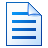ARTICLE TOOLS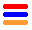Abstract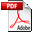PDF Fulltext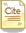How to cite this article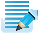Citation Tools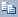Related Records Articles by Nawaf HamadnehArticles by Saratha Sathasivam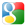on Google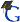on Google Scholar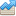Article Statistics Viewed: 1Downloaded: 0